# Steenrod square

(diff) ← Older revision | Latest revision (diff) | Newer revision → (diff)

A stable cohomology operation,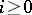, of the type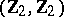, which raises the dimension by. This means that for every integerand every pair of topological spaces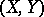, a homomorphism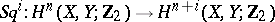is defined such that, whereis the coboundary homomorphism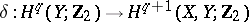(stability) and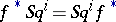for any continuous mapping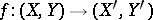(naturality). The Steenrod squares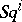possess the following properties:

1);

2)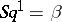, whereis the Bockstein homomorphism associated with the short exact sequence of coefficient groups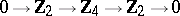;

3) if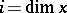, then;

4) if, then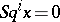;

5) (Cartan's formula)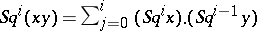;

6) (Adem relation) if, thenwhere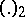are binomial coefficients modulo 2.

In Cartan's formula, multiplication can be considered as outer (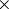-multiplication) as well as interior (cup-multiplication). This is equivalent to the statement that the mapping, defined by the formula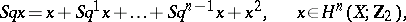is a ring homomorphism. It follows from the stability condition that the Steenrod squarescommute with suspension and transgression.

The properties 1), 3) and 4) define the operationsuniquely and can therefore be taken as defining axioms. The constructive definition ofis based on the simplicial structure in chain groupsand on the existence of a diagonal mapping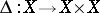. Letbe the minimal acyclic free chain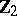-complex i.e. the chain complex for which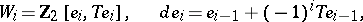whereis the generator of. The existence of an equivariant chain mapping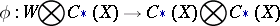such that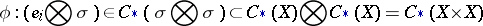for any simplex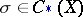, is proved by the method of acyclic carriers or by an explicit construction (see ). The symbolhere signifies the smallest subcomplex of the chain complex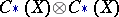containing the element. Let. Any two cochains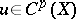,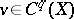are put in correspondence by the formula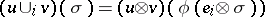, for any simplex, with the cochain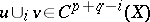, which is called their cup--product. For the coboundary of this chain, the formulaholds, from which it follows that the formulacorrectly defines a homomorphism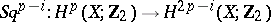which does not depend on the choice of the mapping.

The operationsare constructed in the same way in other simplicial structures with a diagonal mapping, for example, in cohomology groups of simplicial Abelian groups, of simplicial Lie algebras, etc. However, not all properties of the Steenrod squaresare preserved then (for example, generally speaking,) and there is yet (1991) no single general theory for the generalized operations(see , ).

Many cohomology operations which act on cohomology groups with coefficients in the groupsand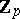can be expressed in terms of the Steenrod squares and their analogues (see Steenrod reduced power). This underlines the fundamental role played by Steenrod squares in algebraic topology and its applications. For example, bordism groups are calculated using Steenrod squares.

Steenrod squares were introduced by N. Steenrod .

How to Cite This Entry:
Steenrod square. Encyclopedia of Mathematics. URL: http://encyclopediaofmath.org/index.php?title=Steenrod_square&oldid=15671
This article was adapted from an original article by S.N. MalyginM.M. Postnikov (originator), which appeared in Encyclopedia of Mathematics - ISBN 1402006098. See original article